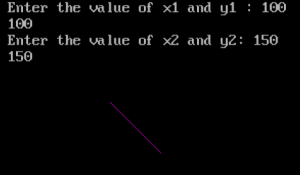# DDA Line Drawing Algorithm in C and C++

Here you will learn about dda line drawing algorithm in C and C++.

In Computer Graphics the first basic line drawing algorithm is Digital Differential Analyzer (DDA) Algorithm.

A line connects two points. It is a basic element in graphics. To draw a line, you need two points between which you can draw a line.

Also Read: Bresenham’s Line Drawing Algorithm in C and C++

### Digital Differential Analyzer (DDA) Algorithm

Step 1: Read the input of the 2 end points of the line as (x1, y1) & (x2, y2) such that x1 != x2 and y1 != y2

Step 2: Calculate dx = x2 – x1 and dy = y2 – y1

Step 3:

if(dx>=dy)

step=dx

else

step=dy

Step 4: xin = dx / step & yin = dy / step

Step 5: x = x1 + 0.5 & y = y1 + 0.5

Step 6:

for(k = 0; k < step; k++)

{

x = x + xin

y = y + yin

putpixel(x, y)

}

## Program for DDA Line Drawing Algorithm in C

Outptut## Program for DDA Line Drawing Algorithm in C++

Comment below if you have any doubts related above algorithm.

### 42 thoughts on “DDA Line Drawing Algorithm in C and C++”

1.No such file or directory
#include
^
compilation terminated.

this error is encountered while compilation of the above written program

1.which compiler you are using?

1.i an using HP computer Intel Pentium inside

1.Dhananjay Panage

2.2.import them for internet

1.Dhananjay Panage

2.yes you right there may be no graphics added in his computer

3.Plz tel me dda line draw algo example

3.3 error on my desktop screen

2.all programs are awesome, Thanks for such a nice blog, where can I get all the commands used in graphics.h ??? I want to know, making circle, and the arguments of circle, what is first one? Or x and y are used to give it postiion, you things like that,

3.Nithyanandhan.V.Gr

Tq, works well

4.A simple way to explain this might be that you’re simply counting the number of ‘steps’ to take between Point 1 and Point 2, calculating the x-axis length and y-axis of each ‘step’, and then looping to add that step length to X and Y respectively for each step between point 1 to point 2.

Unless I’m mistaken, your algorithm here (and your Bresenham algorithm too) only works when X2 > X1 and Y2 > Y1. It’s easy to fix for any two arbitrary points: simply subtract the axis length instead of adding it (dx or dy), in the case that X1 > X2 or Y1 > Y2 respectively.

1.instead of abs in x2-x1 use abs at place of comparing in if statements ,it will work for all cases

5.no error………………output wiil not display…………..

1.change the bgi path

1.how to do that??

2.file>>Change directory >> [set to bgi] >> click Change

1.coder/gamer69

THANK YOU SO MUCH IT WORKED!!!

2.i faced the same problem….

1.change to ‘c://tc//bgi”

6.Bresenham’s algorithm for square

7.{
putpixel(x,y,5);
x=x+dx;
y=y+dy;
i=i+1;
delay(100);
}

ye smjh nhi aaya ki kya use h iska.

1.yah chal nai raha
Enter the value of x1 and y1
Enter the value of x2 and y2
window bgi screen dev pay line show nai ho rahi???????/???

8.graphics.h Error why no such file

1.use turbo c++ instead of other softwares.

2.graphics.h is not available try without Graphics.h and their functions

3.Same Problem There must be no graphics added in your compiler. Import them from internet.

9.THanks a lot brother

10.not running

1.then u run

11.How to run this on macOS or Linux?? The BGI(Borland Graphics Library) was created for Windows. This code doesn’t work on macOS or Linux?

12.after setting path,,,still not displaying output…..

13.declerition not aloted her in gd=DETECT

14.can u tell how run c program in command prompt in win 8……….

1.Dhananjay Panage

First: Go to the working directory (place your file is stored)
Second: open cmd(command promt there) or press Win+r and then enter cmd in the search box then manually change the path (it will be C: by default)
Third: when you are in working directory enter gcc “filename.c” -o “filename.exe” (if it is a c program) if the file compiles correctly then press ./”filename.exe” this will run your code
AND PLEASE MAKE SURE THAT YOU HAVE SET UP YOUR PATH FOR GCC AND REMOVE THE QUOTES WHILE ENTERING THE FILENAME.

15.how to draw multiple lines using dda

16.Hey just checked your code its not applicable for cases like initial points (80,20) and (20,20). Please modify the code

17.Purushottam Prabhakar

can you explain why are you using step 5 with xinc of 0.5 .

18.I got output on the top of window. But according to algorithm output may be shown in x and y axis.

19.your program is not working dude